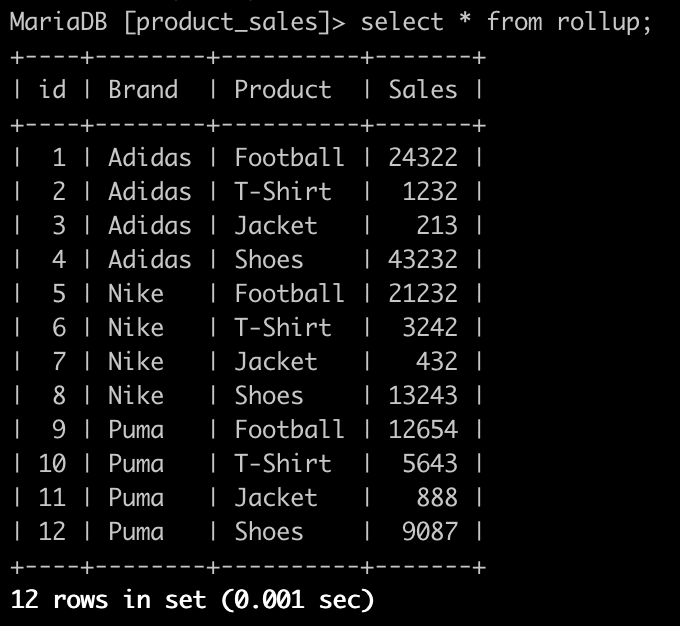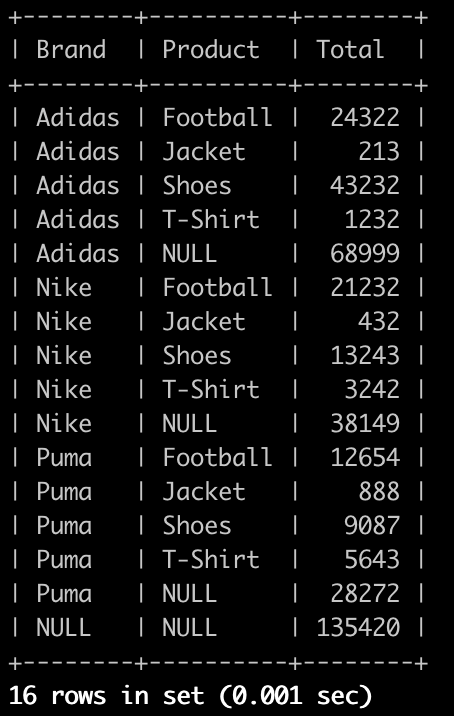Select Page

For our Cube module we use the `ROLLUP` SQL query to fetch all data from the database and then make a tree data structure out of the given result. This is how we make it a lot easier to render cubes dynamically.

What is `ROLLUP`? and how it works?

The SQL `ROLLUP` is a subclause of the GROUP BY clause which provides a shorthand for defining multiple grouping sets.

When generating the grouping sets, `ROLLUP` assumes a hierarchy among the dimension columns and only generates grouping sets based on this hierarchy.

The `ROLLUP` is often used to generate subtotals and totals for reporting purposes.

What does it actually?

To explain this briefly let’s create a SQL table.

```CREATE TABLE rollup
(
id int auto_increment primary key,
Brand varchar(255),
Product varchar(255),
Sales varchar(255)
);```

Now fill some data into the table as follows:

```INSERT INTO rollup (Brand, Product, Sales)
VALUES
('Nike','Football',21232), ('Nike','T-Shirt',3242), ('Nike','Jacket',432), ('Nike','Shoes',13243),
('Puma','Football',12654), ('Puma','T-Shirt',5643), ('Puma','Jacket',888), ('Puma','Shoes',9087);```

The query `SELECT * FROM rollup` should show you the following result:To represent this data in a grouping sets, and to be able to make a tree data structure out of it, we do a simple query: `SELECT Brand, Product, SUM(Sales) AS Total FROM rollup GROUP BY Brand, Product WITH ROLLUP;`

What does this query do?

This gives us the sum for each product of each Brand plus the sum of all Products of a Brand plus the sum of all products of all brands (grand total).

The result will look like the following:To represent this result like a file system tree, we have to create a data tree structure out of this result:

```\$rs = fetchData(); // Database query result
\$columns = ['Brand', 'Products', 'Total'] // Database columns
function getTree(\$rs, \$columns) {
\$pending = new Node();
\$tiers = new SplStack();
\$columns = ['Brand', 'Products', 'Total']

foreach (\$rs as \$data) {
\$data = (object) \$data;

foreach (\$columns as \$dimension) {
if (\$data->\$dimension === null) {
\$pending->setValue(\$data);

while (true) {
if (\$tiers->isEmpty() || \$tiers->top()->getValue()->\$dimension === null) {
break;
}

\$pending->appendChild(\$tiers->pop());
}

\$tiers->push(\$pending);
\$pending = new Node();

continue 2;
}
}
\$pending->appendChild((new Node())->setValue(\$data));
}

\$pending->appendChild(\$tiers->pop());

return \$pending;
}

// Make node recursively iterable
\$iterator = new RecursiveIteratorIterator(getTree(\$rs, \$columns), RecursiveIteratorIterator::SELF_FIRST);

// Print node values recursively
foreach (\$iterator as \$node) {
echo str_repeat(' ', \$iterator->getDepth())
. implode('/', (array) \$node->getValue())
. PHP_EOL;
}```

To make the tree node recursively iterable, pass it to the class `RecurciveIteratorIterator.`

This class needs the passing object to be an instance of traversable, so we have to implement the class `IteratorAggregate` to the `Node` class as following:

```class Node implements IteratorAggregate
{
/** @var Node[] */
protected \$children = [];

/** @var mixed */
protected \$value;

public function hasChildren()
{
return ! empty(\$this->children);
}

/**
* @return Node[]
*/
public function getChildren()
{
return \$this->children;
}

/**
* @param Node \$children
*
* @return \$this
*/
public function appendChild(Node \$child)
{
\$this->children[] = \$child;

return \$this;
}

/**
* @return mixed
*/
public function getValue()
{
return \$this->value;
}

/**
* @param mixed \$value
*
* @return \$this
*/
public function setValue(\$value)
{
\$this->value = \$value;

return \$this;
}

/**
* @inheritDoc
*
* @return NodeIterator
*/
public function getIterator()
{
return new NodeIterator(\$this);
}
}```

Please make sure the class `NodeIterator` implements `RecursiveIteratory` and have the constructor as following:

```public function __construct(Node \$node)
{
\$this->children = new ArrayIterator(\$node->getChildren());
}```

`\$iterator = new RecursiveIteratorIterator(getTree(\$rs, \$columns), RecursiveIteratorIterator::SELF_FIRST);`

The object `\$iterator` can now be iterated recursively.

Have fun experimenting with the rollup iteration and stay tuned for more interesting blog posts.